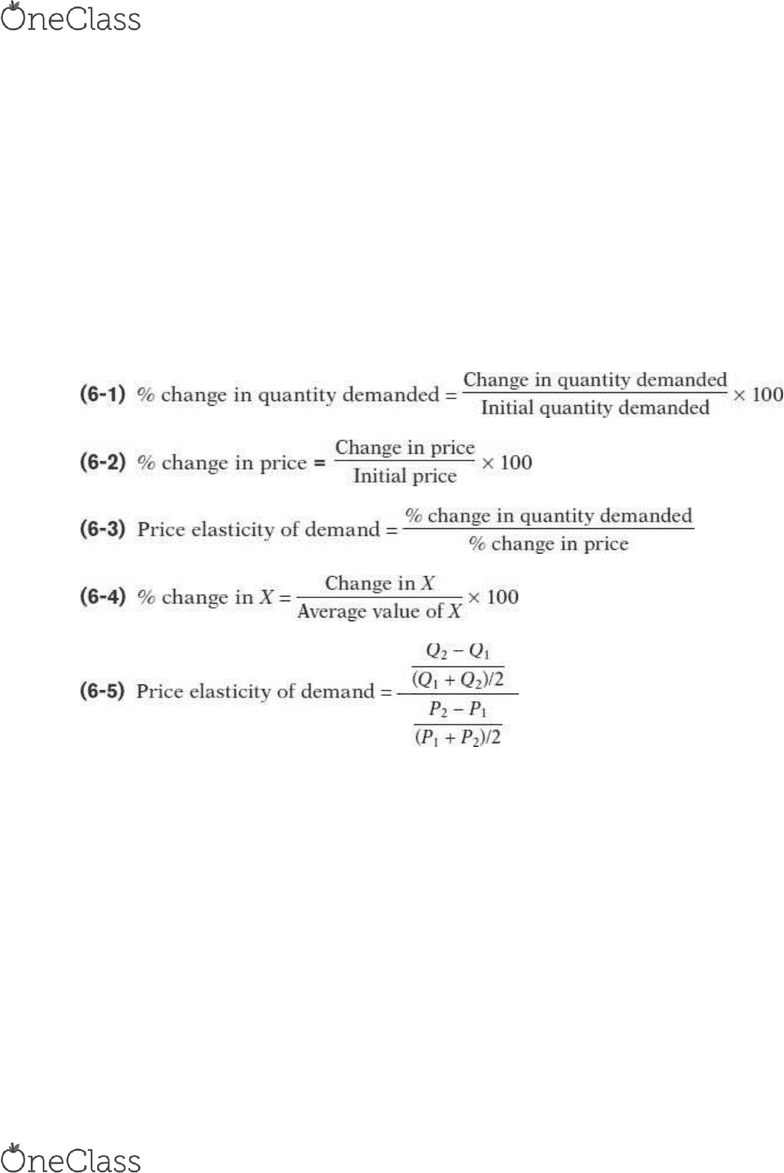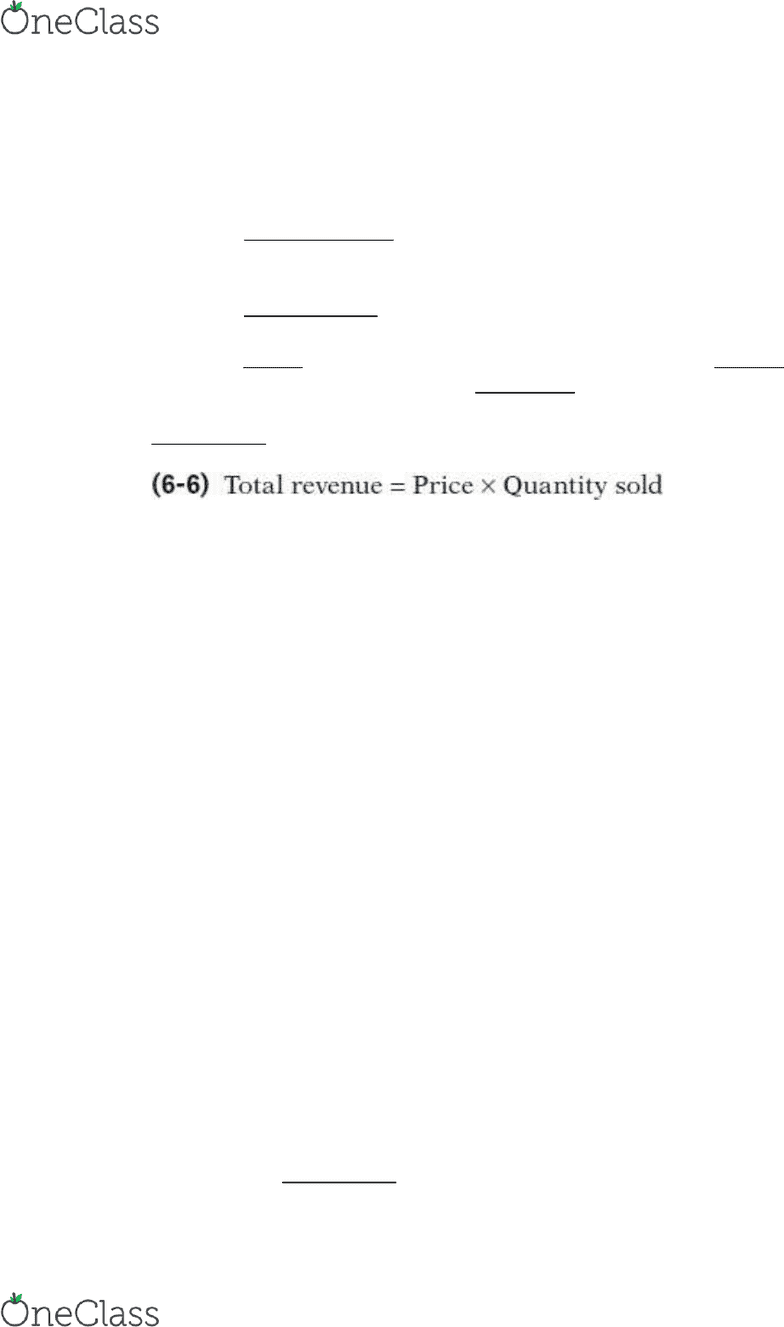Textbook Notes (280,000)
US (110,000)
Rutgers (1,000)
4:40 (200)
Chapter 6

# 01:220:102 Chapter Notes - Chapter 6: Demand Curve, Midpoint Method, Normal Good

Department
Economic
Course Code
01:220:102
Professor
Alex Hohmann
Chapter
6

This preview shows pages 1-2. to view the full 6 pages of the document.Introduction to Microeconomics – 6.1: Defining and Measuring Elasticity
Vocab:
Price elasticity of demand - the ratio of the percent change in the quantity demanded to
the percent change in the price as we move along the demand curve.
Law of demand - demand curves are downward sloping, so price and quantity
demanded always move in opposite directions
Midpoint method - technique for calculating the percent change. In this approach, we
calculate changes in a variable compared with the average, or midpoint, of the starting
and final values
Notes:
Price elasticity of demand compares the percent change in quantity demanded with the
percent change in price.
The price elasticity of demand can be found by comparing the percentage change in
quantity demanded to the percentage change in price
find more resources at oneclass.com
find more resources at oneclass.com

Only pages 1-2 are available for preview. Some parts have been intentionally blurred.Introduction to Microeconomics – 6.2: Interpreting the Price Elasticity of Demand
Vocab
Demand is perfectly inelastic when the quantity demanded does not respond at all to
changes in the price. When demand is perfectly inelastic, the demand curve is a vertical
line
Demand is perfectly elastic when any price increase will cause the quantity demanded
to drop to zero. When demand is perfectly elastic, the demand curve is a horizontal line
Demand is elastic if the price elasticity of demand is greater than 1, inelastic if the price
elasticity of demand is less than 1, and unit-elastic if the price elasticity of demand is
exactly 1
Total revenue - the total value of sales of a good or service. It is equal to the price
multiplied by the quantity sold
Notes:
When a seller raises the price of a good:
A price effect: After a price increase, each unit sold sells at a higher price, which tends to
raise revenue
A quantity effect: After a price increase, fewer units are sold, which tends to lower
revenue
The price elasticity of demand tells us what happens to total revenue when price changes: its
size determines which effect—the price effect or the quantity effect—is stronger.
If demand for a good is unit-elastic (the price elasticity of demand is 1), an increase in
price does not change total revenue. In this case, the quantity effect and the price
effect exactly offset each other.
If demand for a good is inelastic (the price elasticity of demand is less than 1), a higher
price increases total revenue. In this case, the quantity effect is weaker than the price
effect.
If demand for a good is elastic (the price elasticity of demand is greater than 1), an
increase in price reduces total revenue. In this case, the quantity effect is stronger than
the price effect.
4 main factors that determine elasticity:
Whether the good is a necessity or luxury
the availability of close substitutes
the share of income a consumer spends on the good
how much time has elapsed since a change in the price
1. If a change in price causes total revenue to change in the same direction, we can conclude
that the demand is price inelastic
find more resources at oneclass.com
find more resources at oneclass.com